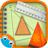MensurationQuantitative Aptitude Questions and Answers section on “Mensuration” with solution and explanation for competitive examinations such as CAT, MBA, SSC, Bank PO, Bank Clerical and other examinations.

The length of a rectangular garden is 12 metres and its breadth is 5 metres. Find the length of the diagonal of a square garden having the same area as that of the rectangular garden :

The length of a rectangular garden is 12 metres and its breadth is 5 metres. Find the length of the diagonal of a square garden having the same area as that of the rectangular garden : [A]$latex 8\sqrt{15} m$ [B]$latex 13 m$ [C]$latex 2\sqrt{30} m$ [D]$latex \sqrt{13} m$ Show Answer $latex \mathbf{2\sqrt{30} m}$ Area of ..

The breadth of a rectangular hall is three-fourth of its length. If the area of the floor is 768 sq. m., then the difference between the length and breadth of the hall is:

The breadth of a rectangular hall is three-fourth of its length. If the area of the floor is 768 sq. m., then the difference between the length and breadth of the hall is: [A]32 meters [B]12 meters [C]8 meters [D]24 meters Show Answer 8 meters Let the length of rectangular hall = x metre ∴ ..

A kite in the shape of a square with a diagonal 32 cm attached to an equilateral triangle of the base cm. Approximately how much paper has been used to make it?

A kite in the shape of a square with a diagonal 32 cm attached to an equilateral triangle of the base 8 cm. Approximately how much paper has been used to make it? [A]$latex 539.217 cm^{2}$ [B]$latex 538.721 cm^{2}$ [C]$latex 540.712 cm^{2}$ [D]$latex 539.712 cm^{2}$ Show Answer $latex 539.712 cm^{2}$ Area of paper = Area ..

From four corners of a square sheet of side 4 cm, four pieces each in the shape of arc of a circle with radius 2 cm, are cut out. The area of the remaining portion is:

From four corners of a square sheet of side 4 cm, four pieces each in the shape of arc of a circle with radius 2 cm, are cut out. The area of the remaining portion is: [A](4 – 2π) sq. cm. [B](16 – 4π) sq. cm. [C](8 – π) sq. cm. [D](16 – 8π) sq. ..

The perimeter of five squares are 24 cm, 32 cm, 40 cm, 76 cm and 80 cm respectively. The perimeter of another square equal in area to sum of the ares of these squares is:

The perimeter of five squares are 24 cm, 32 cm, 40 cm, 76 cm and 80 cm respectively. The perimeter of another square equal in area to sum of the ares of these squares is: [A]62 cm [B]124 cm [C]31 cm [D]961 cm Show Answer 124 cm Side of the squares are 6 cm, 8 ..